# Program to check Magic Number In Java

In this tutorial you will learn about the Program to check Magic Number In Java and its application with practical example.

In this tutorial, we will learn to create a Java Program to check Magic Numbers in Java using Java programming.

## Prerequisites

Before starting with this tutorial we assume that you are best aware of the following Java programming topics:

• Java Operators.
• Basic Input and Output function in Java.
• Class and Object in Java.
• Basic Java programming.
• If-else statements in Java.
• For loop in Java.

## What is Magic Number.

A number is called a Magic number  if sum of its digits repeated calculated till a single digit , If single digit end at 1 then the number is a magic number.

For example:-> 199 is a magic number because 1+9+9 = 19 = 1+9 = 10 = 1+0 = 1.

## Program to check Magic Number In Java

In this program we would find given number is a Magic number or not .first of we would take a value from user and find the Magic number.let have a look at the code

## Output

Magic Number.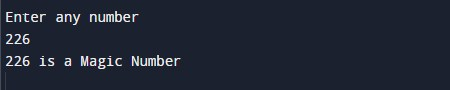Not a Magic Number.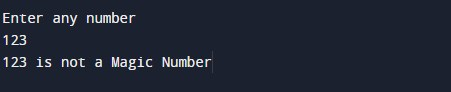In the above program, we have first declared and initialized a set variables required in the program.

• no,number= it will hold entered number.
• Sum=   it will hold result of calculation.

After that we take a number from user and find given number is a Magic number or not.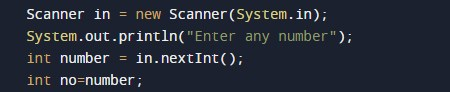Now we will run the loop until(no>0 || sum>9)

• Here we will loop till a single digit is obtained by recursively adding the sum of its digits.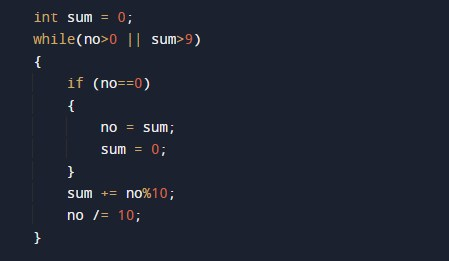• Then we will check last digit of their sum is equals to one (sum==1) if it is one number is a Magic number.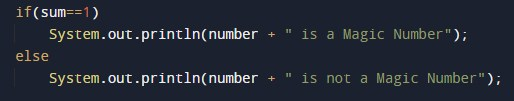• if not it is not a Magic Number.

In this tutorial we have learn about the Program to check Magic Number In Java and its application with practical example. I hope you will like this tutorial.